# Electromagnetic Analysis of Intelligent Reflecting Surface

This example shows how to model the response of an intelligent reflecting surface (IRS) using full-wave electromagnetic simulation. The IRS, also known as the reconfigurable intelligent surface (RIS), large intelligent surface (LIS), or metasurface, has garnered significant interest in the wireless communication community. The IRS consists of a large array of subwavelength unit cell elements which can manipulate the phase, amplitude, polarization, or frequency of the incident signal. If an obstacle blocks the direct path between the base station (BS) and the user equipment (UE), the parasitic reflection of the IRS can provide an alternative path for signal transmission, as this figure shows. An external phase control mechanism can be integrated to control the reflection characteristics of the IRS.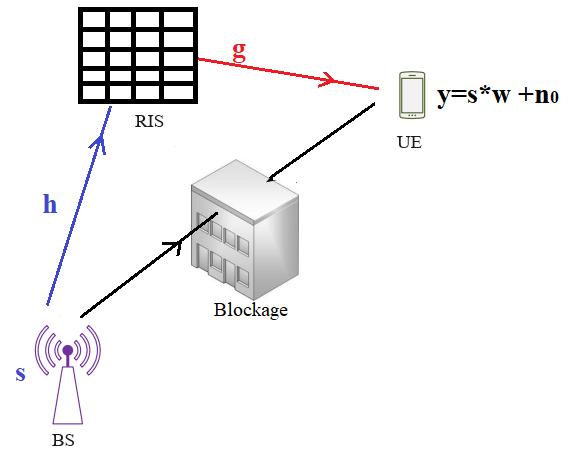The inclusion of the RIS provides a two-segment indirect path to the signal traveling between the BS and the UE. The first segment covers the path between the BS and the IRS. The second segment covers the path between the IRS and the UE. The channel matrix of these two signal paths depends on the geometry and the electromagnetic reflection properties of the IRS.

The signal received at the UE is represented mathematically in  using this equation.

$r=sw+{n}_{0}=s\frac{\sqrt{{G}_{r}{G}_{u}{G}_{t}{A}_{r}{A}_{u}}}{4\pi }\sum _{n=1}^{N}\frac{\sqrt{{\underset{}{\overset{\sim }{F}}}_{n}}R}{{d}_{tn}{d}_{rn}}{e}^{\frac{-j2\pi \left({d}_{tn}+{d}_{rn}\right)}{\lambda }}+{n}_{0}$,

where

• $R=\left[{R}_{1}\phantom{\rule{0.2em}{0ex}}{R}_{2}\phantom{\rule{0.2em}{0ex}}......{R}_{n}{\right]}^{T}.$

• ${G}_{u}$ is the embedded gain of the ${n}^{th}$ IRS element.

• ${G}_{t}$ is the embedded gain of the BS transmitter.

• ${G}_{r}$ is the embedded gain of the UE receiver.

• ${F}_{n}={F}_{n}^{t}{F}_{n}^{r}{F}_{n}^{tx}{F}_{n}^{rx}$ is the product of the normalized power patterns of the BS, UE, and IRS.

• ${d}_{t}$ is the distance between the BS and the ${n}^{th}$ IRS element.

• ${d}_{r}$ is the distance between the UE and the ${n}^{th}$ IRS element.

• ${A}_{r}$ is the effective aperture area of the UE.

• ${A}_{u}$ is the effective aperture area of the ${n}^{th}$ IRS element.

• $\lambda$ is the free-space wavelength.

For this example, assume that the IRS has $N$ unit cell elements. The complex reflection coefficient of the ${n}^{th}$ IRS element is denoted by ${R}_{n}={R}_{n}^{mag}{e}^{j{\tau }_{n}}$.

The reflection coefficients of the IRS depend on these factors:

• Direction and polarization of the incoming signal towards the IRS.

• Direction and polarization of the outgoing signal from the IRS.

• Material properties and geometry of the IRS.

This example focuses on varying the incident signal configurations and determining the corresponding reflection characteristics.

Assume that ${d}_{tn}$ and ${d}_{rn}$ are in the far-field range. You can abstract the IRS as infiniteArray catalog element with a planeWaveExcitation model.

### Assign Operational Frequency

Set the opeational frequency F0 to 5.8 GHz and compute the corresponding wavelength lambda using the speed of light and frequency.

F0 = 5.8e9; lambda = physconst("LightSpeed")/F0; k = 2*pi/lambda;

### Specify Transmitter Location for Incident Wave

Set the location of the transmit source in the far-field region by specifying arbitrary azimuth angle, elevation angle, and radial distance values. To maintain the far-field criterion, the radial distance is 100 times the wavelength. Compute the direction of the incident plane wave signal in the Cartesian coordinate system.

phi_inc = 0; theta_inc = -45:1:45; elevation_inc = 90 + theta_inc; radius_inc = 100*lambda; [x_inc,y_inc,z_inc] = sph2cart(phi_inc*pi/180,elevation_inc*pi/180,radius_inc); inc_loc = [x_inc' y_inc' z_inc'];

### Specify Receiver Location for Reflected Wave

Set the location of observation in the far-field region by specifying arbitrary azimuth angle, elevation angle, and radial distance values. Compute the receiving location in the Cartesian coordinate system.

phi_obs = 0; theta_obs = 20; elevation_obs = 90 - theta_obs; radius_obs = 100*lambda; [x_obs,y_obs,z_obs] = sph2cart(phi_obs*pi/180,elevation_obs*pi/180,radius_obs); obs_loc = [x_obs, y_obs, z_obs];

### Create and Visualize Geometry of Unit Cell

Design a reflector-backed dipole antenna at the operation frequency F0 using the reflector and dipole objects. Tilt the dipole to make it horizontal to the ground plane. Visualize the reflector element using the show function. The ground plane length and reflector width determine the periodicity of the IRS.

rf = design(reflector,F0); rf.Spacing = lambda/10; rf.GroundPlaneLength = 0.5*lambda; rf.GroundPlaneWidth = 0.5*lambda; figure; show(rf)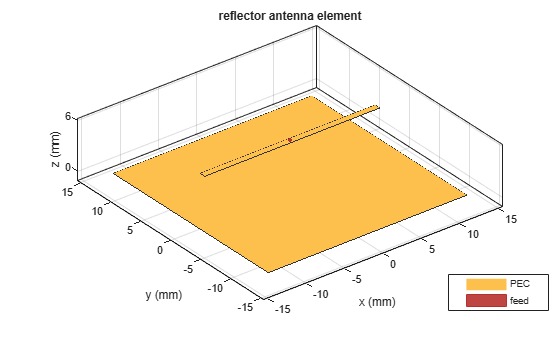### Visualize Finite IRS

Create a 10-by-10 finite IRS using the rectangularArray object and visualize it using the show function. Each unit cell element of the periodic IRS comprises a reflector object with a horizontal bowtie antenna as the exciter.

ifa = rectangularArray(Element=rf, Size=[10 10],... ColumnSpacing=rf.GroundPlaneLength,... RowSpacing=rf.GroundPlaneWidth); figure; show(ifa) title("Finite IRS")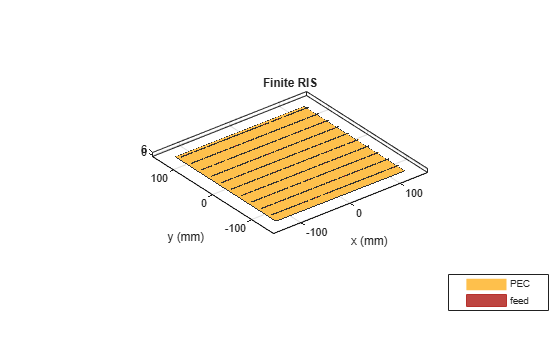### Create and Visualize the Infinite IRS

Commonly, IRS configurations contain a very large number of array elements. Considering the long simulation time of such a large array, infinite array approximation using a periodic Green's function is a suitable alternative. In the Modeling Mutual Coupling in Large Arrays Using Infinite Array Analysis example, you design the IRS using an infiniteArray object.

Assign the reflector as the unit cell element of the IRS. Specify the scan angles of the IRS based on the receiver direction. The accuracy of infinite array modeling depends on the number of summation terms in the periodic Green's function, which you can se using numSummationTerms. Use the show function to visualize the IRS.

irs = infiniteArray(Element=rf); irs.ScanAzimuth = phi_obs; irs.ScanElevation = elevation_obs; numSummationTerms(irs,20); figure; show(irs) title("Infinite IRS")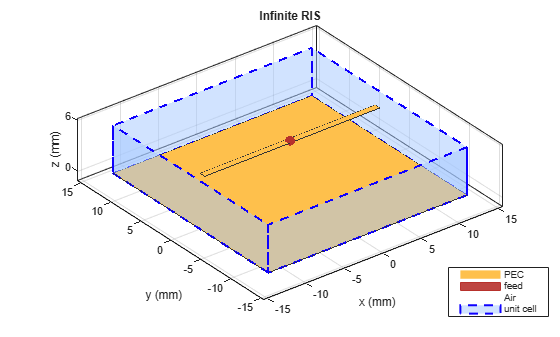% Compute the Array factor AF=hArrayFactorCalc(irs,F0);

### Assign Direction and Polarization to IRS

Initialize the magnitude and phase of the field reflection coefficient for different incident configurations. To excite the IRS, specify it as the Element of planeWaveExcitation object. Specify the polarization and direction of the incident wave.

Initialize the magnitude and phase of the reflection coefficients.

MagReflection=zeros(1,numel(theta_inc)); PhaseReflection=zeros(1,numel(theta_inc)); 

Calculate the reflection coefficients for each value of the incidence angles.

for mm = 1:numel(theta_inc) % Construct a planeWaveExcitation object with the IRS as element [pw,pol,dir] = hcalcPlaneWaveIncidence(theta_inc(mm),phi_inc,irs); % Compute incident field upon the IRS Ein = pol; Einc = dot(Ein,pol/norm(pol)); % Compute the outward scattered field from the IRS [Eo,~] = EHfields(pw,F0,obs_loc'); Eo = Eo*AF; Eobs = dot(Eo,pol/norm(pol)); % Compute the reflection coefficient of the IRS MagReflection(1,mm) = abs(Eobs/Einc); PhaseReflection(1,mm) = (angle(Eobs/Einc))*180/pi; end

### Visualize Reflection Characterstics

Visualize the magnitude and phase of the reflection coefficients. Both the parameters depend on the polarization of incident and reflected signals. Based on the phase of the reflection coefficients, the signal at the receiver end can be constructively or destructively interfered to enhance or reduce the received signal strength, respectively, in a specific observation direction.

figure; plot(theta_inc,MagReflection,"b") title("Reflection Coefficient Magnitude Against Incidence Angle") xlabel("Incidence Angle (\theta_{inc} in degree)") ylabel("Field Reflection Coefficient")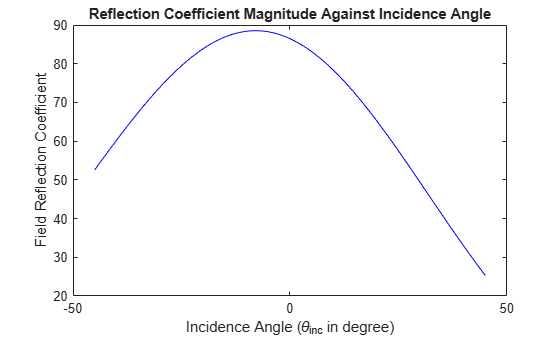figure; plot(theta_inc,PhaseReflection,"b") title("Reflection Coefficient Phase Against Incidence Angle") xlabel("Incidence angle (\theta_{inc} in degree)") ylabel("Field Reflection Coefficient Phase")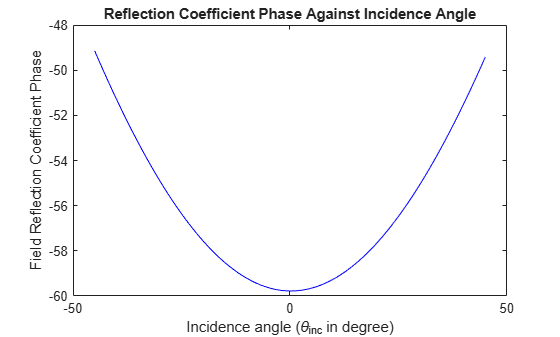You can do this analysis by using alternate infinite array configurations and different unit cell element to achieve desired IRS channel matrix behavior. Based on the phase of the reflection coefficients, the signal at the receiver's end can be constructively/destructively interfered to enhance/reduce the received signal strength in a specific observation direction.

### Further Exploration

Using the full-wave electromagnetic solution of Maxwell's equations from Antenna Toolbox™, you can perform a similar analysis with different IRS configurations constructed from the infiniteArray and planeWaveExcitation objects to design a physics-based smart propagation environment. The geometry of an IRS can be further integrated with Communication Toolbox™ and Optimization Toolbox™ software to obtain an accurate, physics-based characterization of IRS-aided wireless communication scenarios.

### Reference

 Cui, Yaoshen, Haifan Yin, Li Tan, and Marco Di Renzo., " A 3D Positioning-based Channel Estimation Method for RIS-aided mmWave Communications." arXiv:2203.14636 (April 21, 2022). https://doi.org/10.48550/arXiv.2203.14636.